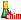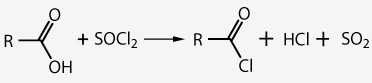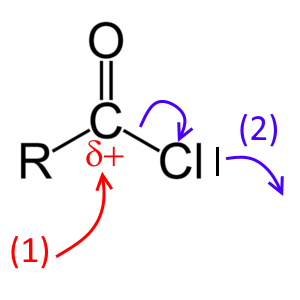﻿ Acid (acyl) chlorides# Acid (acyl) chloridesAcid chloride

## Physical properties

-In contrast to carboxylic acids, they can not form hydrogen bonds between them. Therefore they are more volatile than the corresponding carboxylic acids. - In contact with water, they release $HCl$, hence their lacrymatory effect

## Preparation

### Carboxylic acid and phosporous(V) chloride $PCl_5$

Extract of a video due to Franklychemistry### Carboxylic acid and thionyle chloride $SOCl_2$

Extract of a video due to Franklychemistry## Chemical propertiesThe structure of the functional group of acyl chlorides explains their chemical reactivity:

- (1) The $C$ atom is an electrophilic center ($\delta +$ )

These substances therefore undergo nucleophilic attack, for example by $H_2O^{\delta -}$, $RO^{\delta-}H$, $H_3N^{\delta -}$ or $RH_2N^{\delta -}$:

Reaction with water: $RCOCl + H_2O$ $\rightarrow$ $RCOOH+ HCl$ Product: The corresponding carboxylic acid ! Réaction avec un alcool: $RCOCl + R^{'}OH$ $\rightarrow$ $RCOOR^{'}+ HCl$ Product: The corresponding ester ! Reaction with ammonia: $RCOCl + NH_3$ $\rightarrow$ $RCONH_2+ HCl$ Product: The corresponding non N-substituted amide ! Reaction with an amine: $RCOCl + R^{'}NH_2$ $\rightarrow$ $RCONHR^{'}+ HCl$ Product: The corresponding N-substituted amide !

- (2) The capture of the $Cl$ atom by an electronic gap reagent (eg $AlCl_3$ ) leaves a $+$charge on the $C$ atom

Then these substances can perform an electrophilic attack, for example on a benzene ring: Reaction with benzene in the presence of $AlCl_3$ (Friedel-Crafts reaction): $RCOCl + C_6H_6 + AlCl_3$ $\rightarrow$ $C_6H_5COR+ H^+AlCl_4^-$ Product: The corresponding aromatic carbonyl derivative !

## Examples

Name the end products obtained (by following the above steps) from the reactants: - Methanoyl chloride and isopropanol    →

- Ethanoyl chloride and Methanamine    →

- Methanoyl chloride and benzene (with $AlCl_3$)    →